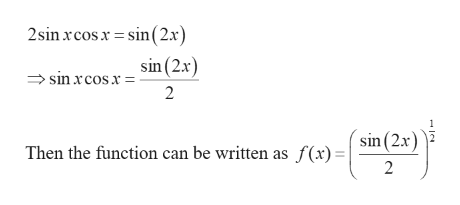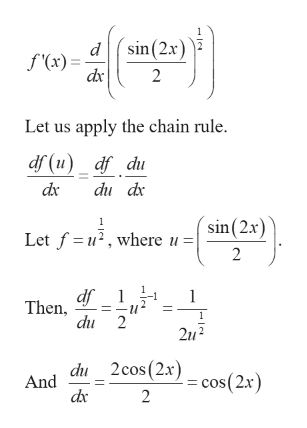# Find the derivative of f(x)=(sinxcosx)1/2

Question
6 views

Find the derivative of f(x)=(sinxcosx)1/2

check_circle

star
star
star
star
star
1 Rating
Step 1

Given the function,

Step 2

We have the identityhelp_outlineImage Transcriptionclose2sin rcosx sin(2x) sin (2x) sin xcosx= 2 sin(2x) Then the function can be written as f(x) = 2 fullscreen
Step 3

Differentiate the ab...help_outlineImage Transcriptionclosed sin (2x) f'(x)= dx 2 Let us apply the chain rule. df (u)_df_du dx du dx sin(2x Let f u, where 2 df Then, 1 1 du 2 2u2 du 2cos(2x) cos(2x) And dic 2 fullscreen

### Want to see the full answer?

See Solution

#### Want to see this answer and more?

Solutions are written by subject experts who are available 24/7. Questions are typically answered within 1 hour.*

See Solution
*Response times may vary by subject and question.
Tagged in

### Derivative